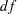## Example 26.3 Testing Uncorrelatedness of Variables

This example uses the sales data in Example 26.1 and tests the uncorrelatedness of the variables in the model by using the MSTRUCT model specification. With the multivariate normality assumption, this is also the test of independence of the variables. The MATRIX statement defines the parameters in the model.

The uncorrelatedness model assumes that the correlations or covariances among the four variables are zero. Therefore, only the four diagonal elements of the covariance matrix, which represent the variances of the variables, are free parameters in the covariance structure model. To specify these parameters, use the MATRIX statement with the MSTRUCT model specification:

```proc calis data=sales;
mstruct var=q1-q4;
matrix _cov_ [1,1], [2,2], [3,3], [4,4];
run;
```

Example 26.1 specifies exactly the same MSTRUCT statement for the four variables. The difference here is the addition of the MATRIX statement. Without a MATRIX statement, the MSTRUCT model assumes that all nonredundant elements in the covariance matrix are model parameters. This assumption is not the case in the current specification. The MATRIX statement specification for the covariance matrix (denoted by the _cov_ keyword) specifies four free parameters on the diagonal of the covariance matrix: [1,1], [2,2], [3,3], and [4,4]. All other unspecified elements in the covariance matrix are fixed zeros by default.

The uncorrelatedness model is displayed in the output for the initial model specification. Output 26.3.1 shows that all off-diagonal elements of the covariance matrix are fixed zeros while the diagonal elements are missing and labeled with _Parm1_Parm4. PROC CALIS generates these parameter names automatically and estimates these four parameters in the analysis.

Output 26.3.1 Initial Uncorrelatedness Model for the Sales Data
Initial MSTRUCT _COV_ Matrix
q1 q2 q3 q4
q1
 . [_Parm1]
 0
 0
 0
q2
 0
 . [_Parm2]
 0
 0
q3
 0
 0
 . [_Parm3]
 0
q4
 0
 0
 0
 . [_Parm4]

Output 26.3.2 shows the model fit chi-square test of the uncorrelatedness model. The chi-square is 6.528 (=6,=0.3667), which is not significant. This means that you fail to reject the uncorrelatedness model. In other words, the data is consistent with the uncorrelatedness model (zero covariances or correlations among the quarterly sales).

Output 26.3.2 Fit Summary of the Uncorrelatedness Model for the Sales Data
Fit Summary
Chi-Square 6.5280
Chi-Square DF 6
Pr > Chi-Square 0.3667

Output 26.3.3 shows the estimates of the covariance matrix under the uncorrelatedness model, together with standard error estimates andvalues. All off-diagonal elements are fixed zeros in the estimation results.

Output 26.3.3 Estimates of Variance under the Uncorrelatedness Model for the Sales Data
MSTRUCT _COV_ Matrix: Estimate/StdErr/t-value
q1 q2 q3 q4
q1
 0.3383 0.1327 2.5495 [_Parm1]
 0
 0
 0
q2
 0
 0.2247 0.0881 2.5495 [_Parm2]
 0
 0
q3
 0
 0
 0.6063 0.2378 2.5495 [_Parm3]
 0
q4
 0
 0
 0
 2.6655 1.0455 2.5495 [_Parm4]

This example shows how to specify free parameters in the MSTRUCT model by using the MATRIX statement. To specify the covariance matrix, use the _COV_ keyword in the MATRIX statement. To specify the parameters in the mean structures, you need use an additional MATRIX statement with the _MEAN_ keyword.

Two important notes regarding the MSTRUCT model specification are now in order:

• When you use the MSTRUCT statement without any MATRIX statements, all elements in the covariance matrix are free parameters in the model (for example, see Example 26.1). However, if the MATRIX statement includes at least one free or fixed parameter in the covariance matrix, PROC CALIS assumes that all other unspecified elements in the covariance matrix are fixed zeros (such as the current example).

• Using parameter names in the MATRIX statement specification is optional. In the context of the current example, naming the parameters is optional because there is no need to refer to them anywhere in the specification. PROC CALIS automatically generates unique names for these parameters. Alternatively, you can specify your own parameter names in the MATRIX statement. Naming parameters is not only useful for references, but is also indispensable when you need to constrain model parameters by referring to their names. See Example 26.4 to use parameter names to define a covariance pattern.